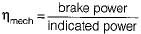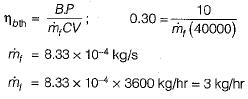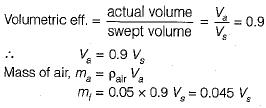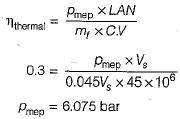Courses

# Test: Basics of IC Engine - 3

## 20 Questions MCQ Test Topicwise Question Bank for Mechanical Engineering | Test: Basics of IC Engine - 3

Description
This mock test of Test: Basics of IC Engine - 3 for Mechanical Engineering helps you for every Mechanical Engineering entrance exam. This contains 20 Multiple Choice Questions for Mechanical Engineering Test: Basics of IC Engine - 3 (mcq) to study with solutions a complete question bank. The solved questions answers in this Test: Basics of IC Engine - 3 quiz give you a good mix of easy questions and tough questions. Mechanical Engineering students definitely take this Test: Basics of IC Engine - 3 exercise for a better result in the exam. You can find other Test: Basics of IC Engine - 3 extra questions, long questions & short questions for Mechanical Engineering on EduRev as well by searching above.
QUESTION: 1

### Engines used for ships are normally

Solution:

Due to low weight, compactness and high power output two-stroke Cl engines are useful in ship propulsion.

QUESTION: 2

### Main advantage of a two-stroke engine over four-stroke engine is

Solution:

Advantages of two-stroke engine over four-stroke engine are
1. Low cost
2. More uniform torque and hence lighter flywheel is needed
3. Because of one power stroke for one revolution, power produced for same size of engine is more
4. Light weight and simplicity due to the absence of valve mechanism.

QUESTION: 3

### For the same indicated work per cycle, mean speed and permissible fluctuation of speed, what is the size of flywheel required for a multi-cylinder engine in comparison to a single-cylinder engine?

Solution:

Torque produced is more uniform in multi- cylinder engine, hence smaller size flywheel is required.

QUESTION: 4

In a four stroke engine the working cycle is completed in

Solution:

In a four-stroke engine the cycle is completed in four stroke of the piston or in two revolution of the crank shaft. Thus one power stroke is obtained in every two revolutions of the crank shaft.

QUESTION: 5

Brake thermal efficiency of the three types of reciprocating engines commonly used in road vehicles are given in the increasing order as

Solution:

Due to scavenging in two-stroke engine brake thermal efficiency of two-stroke engine is less. In Cl engine very high fuel-air. ratio is used as compared to SI engine hence thermal efficiency of Cl engine will be more than SI engine and also in Cl engine higher compressor ratios are used.

QUESTION: 6

Which is not the feature of a petrol engine when compared with a diesel engine

Solution:
QUESTION: 7

Thermal efficiency of Cl engine is higher than that of SI engine due to

Solution:

Since in Cl engines compression ratios are the range of 16-20 while in SI engine, it is of the order of 8-10. Due to higher compression ratio air standard efficiency/thermal efficiency of Cl engine is higher than SI engine.

QUESTION: 8

The volumetric efficiency of the Sl engine is comparatively

Solution:
QUESTION: 9

A two-stroke engine can be identified by

Solution:

QUESTION: 10

Compression ratio in case of SI engine is in the range of

Solution:

For spark ignition engine, compression ratio is in the range of 6-10

QUESTION: 11

Compression ratio in case of Cl engine is in the range of

Solution:

For compression ignition engine, compression ratio is in the range of 16-20

QUESTION: 12

For same power output and same compression ratio, as compared to two-stroke engines, four- stroke SI engine have

Solution:

High fuel consumption, low thermal efficiency and high exhaust temperatures will be associated with 2-stroke engine. 4-stroke engine will have high thermal efficiency because mass flow rate of fuel required will be less in comparison to 2-stroke engine for same power.

QUESTION: 13

Recardo’s theory is associated with

Solution:
QUESTION: 14

Equivalence ratio is

Solution:
QUESTION: 15

Mechanical efficiency is the ratio of

Solution:

Mechanical efficiency,QUESTION: 16

A gas engine has a swept volume of 300 cc and clearance volume of 25 cc. Its volumetric efficiency is 0.88 and mechanical efficiency is 0.90. What is the volume of the mixture taken in per stroke

Solution:

Volumetric efficiency is the ratio of the actual mass of air induced by the engine on the intake stroke to the theoretical mass of air that should have been induced by filling the piston displacement volume with air at atmosphere temperature and pressure.
Volume of mixture taken = 300 x 0.88 = 264 cc

QUESTION: 17

An engine produces 10 kW brake power while working with a brake thermal efficiency of 30%. If the calorific value of the fuel used is 40,000 kJ/kg then what is the fuel consumption?

Solution:QUESTION: 18

An automobile engine operates at a fuel air ratio of 0.05, volumetric efficiency of 90% and indicated thermal efficiency of 30%. Given that the calorific value of the fuel is 45 MJ/kg and the density of air at intake is 1 kg/m3, the indicated mean effective pressure for the engine is

Solution:QUESTION: 19

In a SI engine very high compression ratio cannot be used because

Solution:
QUESTION: 20

For the following “Matching” exercise, choose the correct one from among the alternatives a, b, c and d
Group-I
1. Marine Diesel Engine
2. Air-conditioning
3. Steam power plant
4. Gas turbine power plant
Group-II
(a) Two stroke engine
(b) Four stroke engine
(c) Rotary engine
(d) Cooling and dehumidification
(e) Cooling tower
(f) Brayton cycle
(g) Rankine cycle
(h) D-slide valve

Solution:

Two stroke engine ⇒ used in marine diesel engine.
Air conditioning ⇒ Cooling and dehumidification is done.
Steam power plant ⇒ Based on Rankine cycle.
Gas turbine power plant ⇒ Based on Brayton cycle